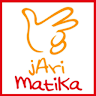# Jarimatika (short fingers and Mathematics)

## Information

 Package com.tipsdani.jarimatika.berhitungmetodejarimatika.creativedeveloper.jayaraya.jarimatika Version 4.0 (4) Updated 2019-08-07 Size 10 MB Installs 1,000 Category Education

## Description

Jarimatika (Finger of Mathematics)

Jarimatika (short for Jari and Mathematics) is a way of counting either the sum, subtraction, division or multiplication of mathematics by using the fingers. With the application of Jarimatika (Finger Mathematics) Complete - Smart and Fast counting is expected to students or teachers can perform math counting operations that is the addition, subtraction, multiplication and sharing use our fingers.

Know our fingers is a miracle, which can be used to complete multiplication without a calculator.

Jarimatika (Finger of Mathematics) Complete - Smart and Fast Counting is a MAGIC application that is one of the breakthroughs in the world of mathematics.

Hopefully this can be useful for pupils and teachers in teaching multiplication for the students.

Jarimatika App (Mathematical Finger) - Intelligent and Quick Methods Count using Finger.

Jarimatika (Finger of Mathematics) Complete, Latest and Update

Do not Forget Love 5 Stars yes. Thank you

Jarimatika (short fingers and Mathematics) is counting math for kids by using your fingers developed by Mr. Dani.

Know our fingers is a miracle, which can be used to solve multiplication without a calculator.

This is an application Jarimatika MAGIC which is one of the breakthroughs in the world of mathematics.

Hopefully this can be beneficial for teachers to teach multiplication for students.

## What's New

Lighter and simpler